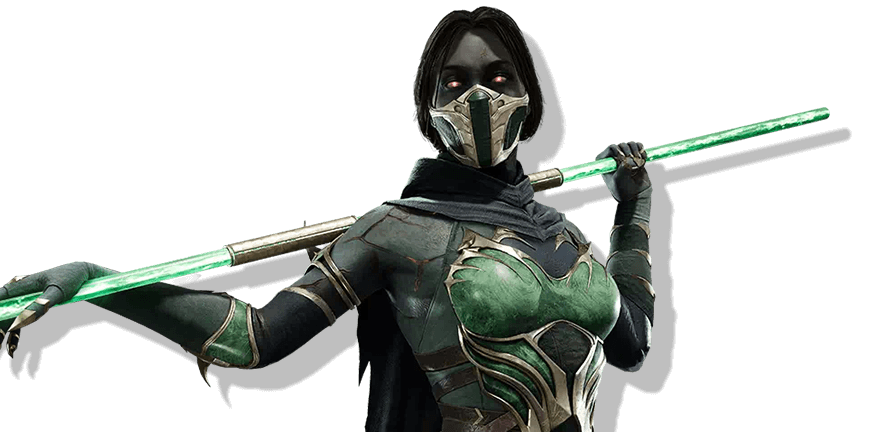Start learning movesets and combos!

Choose the platform you play on.
See how to read and perform inputs.Mortal Kombat 11

Jade quickly became a very charismatic MK character. Her sarcastic and patronizing attitude has earned her a few fans accolades. She is loyal to her allies and is willing to fight for her ideals. Jade is a fierce female Fighter and assassin.Know more

Get notified of the character’s updates

## Basic combo attacks

Combinations of basic moves

One
,
Two

One
,
Two
,
Four

Back
+
One
,
Two

Two
,
One

Two
,
One
,
Two

Forward
+
Two
,
One

Back
+
Three
,
Four
,
Three

Back
+
Three
,
Four
,
Three
,
Four

Forward
+
Three
,
Four

Forward
+
Three
,
Four
,
Three

Four
,
Three

### My Pleasure

Forward
+
Four
,
One

Long combinations of various moves including abilities and specials

One
,
Two
,
Four
KB
Forward
Forward
Four
Down
Back
One
AMP
Down
+
Two

Forward
+
One
+
Three
KB
Forward
Forward
Four
Back
Forward
AMP
Down
+
Two

Up
+
Two
Forward
Forward
Two
Back
Forward
One
AMP
Down
+
Two

Down
+
Two
Forward
Forward
Two
,
One
Back
+
Two
Down
Forward
Four

Forward
+
Two
,
One
Forward
Forward
Four
Back
Forward
One
AMP
Down
+
Two

Forward
+
Two
,
One
Back
+
Two
FB

One
,
Two
,
Four
KB
Four
Back
Forward
Three

Forward
+
One
+
Three
KB
Forward
Forward
Four
Back
Forward
One
AMP
Down
+
Two

Up
+
Two
Forward
Forward
Two
Back
Forward
One
AMP
Down
+
Two

Down
+
Two
KB
Forward
Forward
Two
Back
Forward
One
AMP
Down
+
Two

Forward
+
Two
,
One
Forward
Forward
Four
Back
Forward
One
AMP
Down
+
Two

Forward
+
Two
,
One
Back
+
Two
FB

One
,
Two
,
Four
KB
Forward
Forward
Three
,
Two
,
Two
,
Down
+
One

Forward
+
One
+
Three
KB
Forward
Forward
Four
Back
Forward
One
AMP
Down
+
Two

Up
+
Two
Forward
Forward
Two
Back
Forward
One
AMP
Down
+
Two

Down
+
Two
KB
Forward
Forward
Two
Back
Forward
One
AMP
Down
+
Two

Down
Back
Two
AMP
Three
,
Two
,
Two
,
Down
+
One

Forward
+
Two
,
One
Forward
Forward
Four
Back
Forward
One
AMP
Down
+
Two

Forward
+
Two
,
One
Back
+
Two
FB

Down
+
Two
KB
Forward
Forward
Two
Back
Forward
One
AMP
Down
+
Two

Forward
+
Two
,
One
Forward
Forward
Four
Back
Forward
One
AMP
Down
+
Two

Forward
+
Two
,
One
Back
+
Two
FB

Up
+
Two
Forward
Forward
Two
,
One
Back
Forward
One
AMP
Down
+
Two

Forward
+
One
,
Three
KB
Forward
Forward
Three
,
Two
,
Two
,
Down
+
One